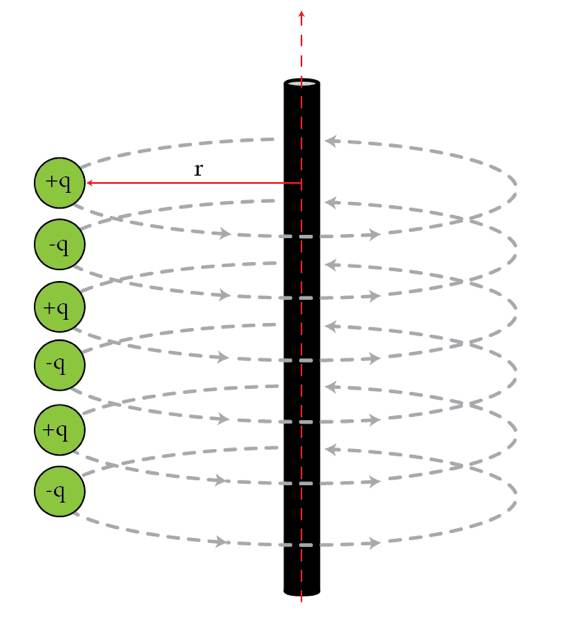# Correlations and temperature

Phase transitions, like from liquid to solid, are familiar in everyday experience. More exotic phase transitions, such as the change of a conductor to a superconductor, are characterized by a "correlation length." The correlation length is the length over which properties of the system look the same.

As a simple example, consider a line of alternating charges ($+q$ and $-q$), connected by small nonconducting rods to a long vertical rod, as in the figure below. In cylindrical coordinates the charges are at $r=R, z=\ldots -2,-1,0,1,2 \ldots$ and can only rotate around the long rod, i.e. they can only move in $\theta$. At absolute zero, none of the charges can ever have any kinetic energy. Therefore they must all be in their lowest energy state, with all the small rods aligned. Hence for absolute zero the correlation length is infinity, in that if I measure the angle $\theta$ of one rod, I know the angle $\theta$ for a rod an infinite distance away.

If we increase the temperature, then the rods will start to wiggle back and forth. At very high temperatures, the rods are spinning around like mad, and so measuring the angle $\theta$ for one rod tells us nothing about the rod right next to it. In this case the correlation length is zero. The phase transition in this situation is from an uncorrelated or disordered system at non-zero temperature, to a completely ordered system at absolute zero.

Correlation lengths generically diverge with temperature as $L\propto |T-T_c|^{-\nu}$, where $T_c$ is a critical temperature (in our case absolute zero for simplicity), $T$ is near $T_c$ and $\nu$ is a positive number. If we define $L$ for our case as the minimum number of rods between two rods that are oppositely aligned, what is $\nu$ for very low temperatures?This problem is in memoriam of Kenneth Wilson, who developed much of what we know about phase transitions and passed away two weeks ago.

Details and assumptions

• For simplicity, only consider nearest neighbor interactions between the charges.
• Ignore gravity.
×

Problem Loading...

Note Loading...

Set Loading...